# CLASS 12 MATHS CHAPTER 6-APPLICATION OF DERIVATIVES

### Solve The Following Questions.

Question1. Find the rate of change of the area of a circle with respect to its radius r when

(a)r  = 3 cm

(b)r = 4 cm

Solution :
The area of a circle (A) with radius (r) is given by,

A = πr2

Now, the rate of change of the area with respect to its radius is given by,
=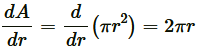(a) When r = 3 cm, then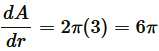sq. cm

(b) When r = 4 cm, then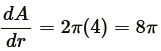sq. cm

Question2. The volume of a cube is increasing at the rate of 8 cm3/sec. How fast is the surface area increasing when the length of an edge is 12 cm?

Solution :
Let x  be the length of a side,  V be the volume, and s be the surface area of the cube.

Given: Rate of increase of volume of cube = 8 cm3/sec

Then, V = x3 and S = 6x2 where x is a function of time t

It is given tha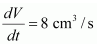Hence, if the length of the edge of the cube is 12 cm, then the surface area is increasing at the rate of 8/3 cm2/s.

Question3. The radius of the circle is increasing uniformly at the rate of 3 cm per second. Find the rate at which the area of the circle is increasing when the radius is 10 cm.

Solution :

The area of a circle (A) with radius (r) is given by,

A = πr2

Now, the rate of change of the area with respect to its radius is given by,
=It is given that,

Hence, the rate at which the area of the circle is increasing when the radius is 10 cm is 60π cm2/s.

Question4. An edge of a variable cube is increasing at the rate of 3 cm per second. How fast is the volume of the cube increasing when the edge if 10 cm long?

Solution :

Let x be the length of a side and V be the volume of the cube. Then,

V = x3.

Hence, the volume of the cube is increasing at the rate of 900 cm3/s when the edge is 10 cm long.

Question5. A stone is dropped into a quite lake and waves move in circles at the rate of 5 cm/sec. At the instant when radius of the circular wave is 8 cm, how fast is the enclosed area increasing?

Solution:

The area of a circle (A) with radius (r) is given by

A = πr2

Therefore, the rate of change of area (A) with respect to time (t) is given by,

Hence, when the radius of the circular wave is 8 cm, the enclosed area is increasing at the rate of 80π cm2/s.

Question6. The radius of a circle is increasing at the rate of 0.7 cm/s. What is the rate of its circumference?

Solution :

The circumference of a circle (C) with radius (r) is given by

C = 2πr.

Therefore, the rate of change of circumference (C) with respect to time (t) is given by,

Hence, the rate of increase of the circumference is 2π(0.7) = 1.4π cm/s

Question7. The length x of a rectangle is decreasing at the rate of 5 cm/minute. When x = 8 cm and y = 6 cm, find the rates of change of (a) the perimeter and (b) the area of the rectangle.

Solution :

Since the length (x) is decreasing at the rate of 5 cm/minute and the width (y) is increasing at the rate of 4 cm/minute, we have:

Hence, the area of the rectangle is increasing at the rate of 2 cm2/min.

Question8. A balloon, which always remains spherical on inflation, is being inflated by pumping in 900 cubic centimeters of gas per second. Find the rate at which the radius of the balloon increases when the radius is 15 cm.

Solution :

The volume of a sphere (V) with radius (r) is given by,

V = 4/3πr3

∴Rate of change of volume (V) with respect to time (t) is given by,

Hence, the rate at which the radius of the balloon increases when the radius is 15 cm is 1/π cm/s.

Question9. A balloon, which always remains spherical has a variables radius. Find the rate at which its volume is increasing with the radius when the later is 10 cm.

Solution :
Since, V = 4/3πr3

Rate of change of volume (V) with respect to its radius (r) is given by,

Hence, the volume of the balloon is increasing at the rate of 400π cm2.

Question10. A ladder 5 cm long is leaning against a wall. The bottom of the ladder is pulled along the ground, away from the wall, at the rate of 2 cm/s. How fast is its height on the wall decreasing when the foot of the ladder is 4 m away from the wall?

Solution :

Let y m be the height of the wall at which the ladder touches. Also, let the foot of the ladder be maway from the wall.

Then, by Pythagoras theorem, we have:

x2 + y2 = 25 [Length of the ladder = 5 m]

⇒ y = √25 – x2

Then, the rate of change of height (y) with respect to time (t) is given by,

Hence, the height of the ladder on the wall is decreasing at the rate of 8/3 cm/s.

Question 11. A particle moves along the curve 6y = x3 + 2 Find the points on the curve at which the y-coordinate is changing 8 times as fast as the x-coordinate.

Solution :
Given: Equation of the curve  6y = x3 + 2……….(i)

The rate of change of the position of the particle with respect to time (t) is given by,

Question12. The radius of an air bubble is increasing at the rate of 1/2 cm/s. At what rate is the volume of the bubble increasing when the radius is 1 cm?

Solution :

The air bubble is in the shape of a sphere.

Now, the volume of an air bubble (V) with radius (r) is given by,

V = 4/3πr3

The rate of change of volume (V) with respect to time (t) is given by,

Hence, the rate at which the volume of the bubble increases is 2π cm3/s.

Question13. A balloon which always remains spherical, has a variable diameter 3/2 (2x + 1) Find the rate of change of its volume with respect to x

Solution :

The volume of a sphere (V) with radius (r) is given by,

V = 4/3πr3

It is given that:

Diameter 3/2 (2x + 1)

Question14. Sand is pouring from a pipe at the rate of 12 cm3/s. The falling sand forms a cone on the ground in such a way that the height of the cone is always one-sixth of the radius of the base. How fast is the height of the sand cone increasing when the height is 4cm?

Solution :

The volume of a cone (V) with radius (r) and height (h) is given by,

V = 4/3πr3

It is given that,

h = 1/6r ⇒r = 6h

Hence, when the height of the sand cone is 4 cm, its height is increasing at the rate of 1/48π cm/s.

Question15. The total cost C(x) in rupees associated with the production of x units of an item given by  Find the marginal cost when 17 units are produced.

Solution :

Marginal cost is the rate of change of total cost with respect to output.

Hence, when 17 units are produced, the marginal cost is Rs. 20.967.

Question16. The total revenue in rupees received from the sale of x units of a product is given by

Find the marginal revenue when x = 7

Solution :
Marginal Revenue (MR) =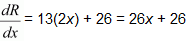When x = 7,

MR = 26(7) + 26 = 182 + 26 = 208

Hence, the required marginal revenue is Rs 208.

Choose the correct answer in Exercises 17 and 18.

Question17. The rate of change of the area of a circle with respect to its radiusr at r = 6 cm is

(A) 10π

(B) 12π

(C) 8π

(D) 11π

Solution :

The area of a circle (A) with radius (r) is given by,

A = πr2

Therefore, the rate of change of the area with respect to its radius r is

Hence, the required rate of change of the area of a circle is 12π cm2/s.

Question18. The total revenue in Rupees received from the sale of x units of a product is given by  R(x) = 3x2 + 36x + 5 The marginal revenue, when x = 15 is:

(A) 116

(B) 96

(C) 90

(D) 126

Solution :

Marginal revenue is the rate of change of total revenue with respect to the number of units sold.

∴Marginal Revenue (MR)= dR/dx= 3(2x) + 36 = 6x + 36

∴When x = 15,

MR = 6(15) + 36 = 90 + 36 = 126

Hence, the required marginal revenue is Rs 126.

### Exercise 6.2

Solve The Following Questions.

Question1. Show that the function given by f(x) = e2x  is strictly increasing on R.

Solution :
Given: f'(x) = e2x

Now,

x ∈ R

Since the value of  e2x   is always positive for any real value of x, e2x > 0.

⇒2e2x > 0

⇒f'(x) > 0

So f(x) is incerasing on R.

Question2. Show that the function given by f(x) = 3x + 17 is strictly increasing on R.

Solution :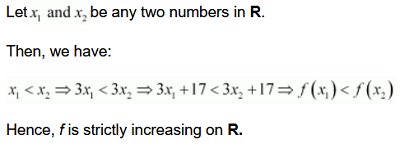Question3. Show that the function given by f(x) = sin x is

(a) strictly increasing (0,π/2) (b) strictly decreasing in (π/2,π)  (c) neither increasing nor decreasing in (0,π)

Solution :
Given:

The given function is f(x) = sin x.

Question4. Find the intervals in which the function  F given by 2x2 – 3x  is (a) strictly increasing, (b) strictly decreasing.

Solution :
Given:

Question5. Find the intervals in which the function F given by f(x) = 2x3 − 3x2 − 36x + 7 is (a) strictly increasing, (b) strictly decreasing.

Solution :
(a)Given:

Question6. Find the intervals in which the following functions are strictly increasing or decreasing:

(a) x2 + 2x − 5 (b) 10 − 6x − 2x2

(c) −2x3 − 9x2 − 12x + 1 (d) 6 − 9x − x2

(e) (x + 1)3 (x − 3)3

Solution :

(a) Given: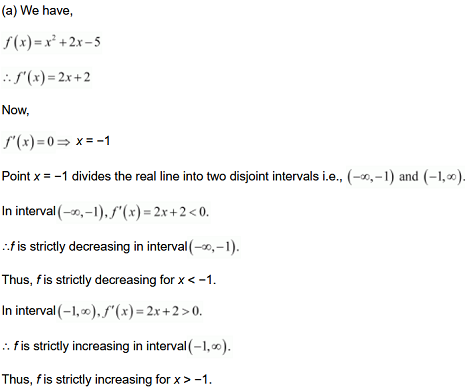Question7. Show that   is an increasing function of x throughout its domain.

Solution :
Given: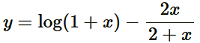∴dydx=11+x-(2+x)(2)-2x(1)(2+x)2=11+x-4(2+x)2=x2(1+x)(2+x)2

Now, dydx=0

⇒x2(1+x)(2+x)2=0⇒x2=0      [(2+x)≠0 as x>-1]⇒x=0

Since x > -1 , point = 0 divides the domain (−1, ∞) in two disjoint intervals i.e., −1 < x  < 0 and x > 0

When −1 < x < 0, we have:

x<0⇒x2>0x>-1⇒(2+x)>0⇒(2+x2)>0

∴ y’=x2(1+x)(2+x)2>0

Also, when x > 0

x>0⇒x2>0, (2+x)2>0

∴ y’=x2(1+x)(2+x)2>0

Hence, function f  is increasing throughout this domain

Question8. Find the value of x  for which   is an increasing function.

Solution :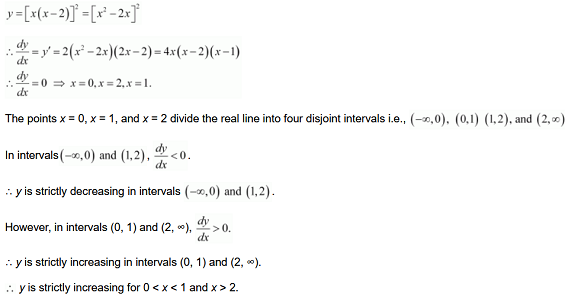Question9. Prove that   is an increasing function of θ  in [0, π/2]

Solution :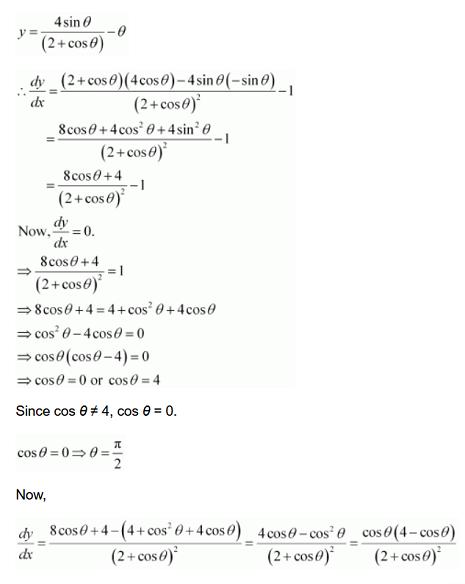Question10. Prove that the logarithmic function is strictly increasing on (0,∞)

Solution :
Given: The given function is f(x) =  logx

∴ f'(x) = 1/x

It is clear that for x > 0,  f'(x) = 1/x > 0.

Hence, f(x) = log x  is strictly increasing in interval (0, ∞).

Question11. Prove that the function f  given by f (x) = x2 – x + 1  is neither strictly increasing nor strictly decreasing on (-1,1)

Solution :
Given: The given function f (x) = x2 – x + 1

hence,f is neither strictly increasing nor decreasing on the interval (-1,1)

Question12. Which of the following functions are strictly decreasing on (0,π/2)

(A) cos x

(B) cos 2x

(C) cos 3x

(D) tan x

Solution :

Question13. On which of the following intervals is the function f given by f(x) = x100 + sinx – 1 is strictly decreasing:

(A) (0, 1)

(B) (π/2,π)

(C) (0,π/2)

(D) None of these

Solution :
Given:

Question14. Find the least value of a  such that the function f given by f(x) = x2 + ax + 1 strictly increasing on (1, 2).

Solution :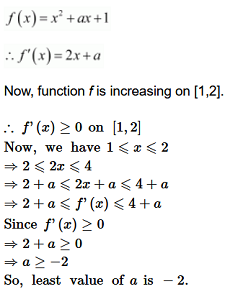Question15. Let I be any interval disjoint from (-1,1)  Prove that the function f given by f(x) = x + 1/x  is strictly increasing on I.

Solution :
Given:

∴ f is strictly increasing on (-∞, 1) and (1, ∞)

Hence, function f  is strictly increasing in interval  I  disjoint from (−1, 1).

Hence, the given result is proved.

Question16. Prove that the function f given by f(x) = log sin x is strictly increasing on (0,π/2) and strictly decreasing on (π/2,π)

Solution :
Given:

Question17. Prove that the function f given by f(x) = log cos x is strictly decreasing on (0,π/2) and strictly decreasing on (π/2,π)

Solution :
Given:

On the

Question18. Prove that the function given by f(x) = x3 – 3x2 + 3x – 100 is increasing in R.

Solution :
Given:

Question19. The interval in which y = x2 e-x  is increasing in:

(A) (-∞, ∞)

(B)(-2,0)

(C) (2, ∞)

(D) (0, 2)

Solution :
Given:

### Solve The Following Questions.

Question1. Find the slope of the tangent to the curvey = 3x4 − 4x at x = 4.

Solution :
The given curve isy = 3x4 − 4x.

Then, the slope of the tangent to the given curve at x = 4 is given by,

Question2. Find the slope of the tangent to the curve,x ≠ 2 at x = 10.

Solution :
The given curve is .

Hence, the slope of the tangent at x = 10 is -1/64

Question3. Find the slope of the tangent to curve y =x3 −x + 1 at the point whose x-coordinate is 2.

Solution :
The given curve is y =x3 −x + 1

Question4. Find the slope of the tangent to the curve y =x3 − 3x + 2 at the point whose x-coordinate is 3.

Solution :
The given curve is y =x3 − 3x + 2

Question5. Find the slope of the normal to the curve x =a cos3θ, y = a sin3θ at  θ = π/4.

Solution :
It is given that x =a cos3θ and y =a sin3θ.

Question6. Find the slope of the normal to the curvex = 1 − a sinθ, y =b cos2θ at θ = π/2.

Solution :
It is given thatx = 1 −a sinθ and y =b cos2θ.

Question7. Find points at which the tangent to the curve y =x3 − 3x2 − 9x + 7 is parallel to thex-axis.

Solution :
The equation of the given curve is y =x3 − 3x2 − 9x + 7

When x = 3, y = (3)− 3 (3)2 − 9 (3) + 7 = 27 − 27 − 27 + 7 = −20.

When x = −1,y = (−1)3 − 3 (−1)2 − 9 (−1) + 7 = −1 − 3 + 9 + 7 = 12.

Hence, the points at which the tangent is parallel to thex-axis are (3, −20) and (−1, 12).

Question8. Find a point on the curvey = (x − 2)2 at which the tangent is parallel to the chord joining the points (2, 0) and (4, 4).

Solution :
If a tangent is parallel to the chord joining the points (2, 0) and (4, 4), then the slope of the tangent = the slope of the chord.

The slope of the chord is 4-0/4-2 = 4/2 = 2

Now, the slope of the tangent to the given curve at a point (x,y) is given by,

Hence, the required point is (3, 1).

Question9. Find the point on the curve y =x3 − 11x + 5 at which the tangent is y =x − 11.

Solution :
The equation of the given curve is y =x3 − 11x + 5.

The equation of the tangent to the given curve is given as y =x − 11 (which is of the form y =mx +c).

∴Slope of the tangent = 1

Now, the slope of the tangent to the given curve at the point (x,y) is given by, dy/dx = 3x2 – 11

Then, we have:

Whenx = 2,y = (2)3 − 11 (2) + 5 = 8 − 22 + 5 = −9.

Whenx = −2,y = (−2)3 − 11 (−2) + 5 = −8 + 22 + 5 = 19.

Hence, the required points are (2, −9) and (−2, 19). But, both these points should satisfy the equation of the tangent as there would be point of contact between tangent and the curve.

∴ (2, −9) is the required point as (−2, 19) is not satisfying the given equation of tangent.

Question10.  Find the equation of all lines having slope −1 that are tangents to the curve y = 1/x-1 ≠ 1

Solution :
The equation of the given curve is y = 1/x-1 ≠ 1

The slope of the tangents to the given curve at any point (x,y) is given by,

dy/dx = -1/(x-1)2

If the slope of the tangent is −1, then we have:

Whenx = 0,y = −1 and when x = 2,y = 1.

Thus, there are two tangents to the given curve having slope −1. These are passing through the points (0, −1) and (2, 1).

∴The equation of the tangent through (0, −1) is given by,

y − (-1 )= −1 (x − 0)

⇒y +1 = −x

⇒y +x +1 = 0

∴The equation of the tangent through (2, 1) is given by,

y − 1 = −1 (x − 2)

⇒y − 1 = −x + 2

⇒y +x − 3 = 0

Hence, the equations of the required lines are y +x + 1 = 0 and y +x − 3 = 0.

Question11. Find the equation of all lines having slope 2 which are tangents to the curve y = 1/x-3 ≠ 3

Solution :
The equation of the given curve is y = 1/x-3 ≠ 3

The slope of the tangent to the given curve at any point (x,y) is given by,

Hence, there is no tangent to the given curve having slope 2.

Question12. Find the equations of all lines having slope 0 which are tangent to the curve.

Solution :
The equation of the given curve is

The slope of the tangent to the given curve at any point (x,y) is given by,

Question13. Find points on the curveat which the tangents are

(i) parallel tox-axis

(ii) parallel toy-axis

Solution :
The equation of the given curve is

On differentiating both sides with respect to x, we have:

Hence, the points at which the tangents are parallel to they-axis are  (3, 0) and (− 3, 0).

Question14. Find the equations of the tangent and normal to the given curves at the indicated points:

(i) y =x4 − 6x3 + 13x2 − 10x + 5 at (0, 5)

(ii) y =x4 − 6x3 + 13x2 − 10x + 5 at (1, 3)

(iii) y =x3 at (1, 1)

(iv) y =x2 at (0, 0)

(v) x = cost,y = sint at ,t = π/4

Solution :
(i) The equation of the curve is y = x4 − 6x3 + 13x2 − 10x + 5.

On differentiating with respect tox, we get:

(ii) The equation of the curve is y = x4 − 6x3 + 13x2 − 10x + 5.

On differentiating with respect tox, we get:

(iii) The equation of the curve isy =x3.

On differentiating with respect tox, we get:

(iv) The equation of the curve isy =x2.

On differentiating with respect tox, we get:

(v) The equation of the curve is x = cost, y = sint.

Question15. Find the equation of the tangent line to the curve y = x2 − 2x + 7 which is

(a) parallel to the line 2x −y + 9 = 0

(b) perpendicular to the line 5y − 15x = 13.

Solution :
The equation of the given curve is y = x2 − 2x + 7

On differentiating with respect tox, we get:

dy/dx = 2x – 2

(a) The equation of the line is 2x −y + 9 = 0.

2x −y + 9 = 0⇒y = 2x+ 9

This is of the formy = mx+c.

∴Slope of the line = 2

If a tangent is parallel to the line 2x −y + 9 = 0, then the slope of the tangent is equal to the slope of the line.

Therefore, we have:

2 = 2x − 2

2x = 4

x = 2

Now,x = 2

y = 4 − 4 + 7 = 7

Thus, the equation of the tangent passing through (2, 7) is given by,

y – 7 = 2(x – 2)

y – 2x – 3 = 0

Hence, the equation of the tangent line to the given curve (which is parallel to line 2x −y + 9 = 0) is y – 2x – 3 = 0.

(b) The equation of the line is 5y − 15x = 13.

5y − 15x = 13⇒ y = 3x + 13/5

This is of the formy =mx+c.

∴Slope of the line = 3

If a tangent is perpendicular to the line 5y − 15x = 13, then the slope of the tangent is -1/slope of the line = -1/3

Thus, the equation of the tangent passing through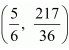is given by,

Hence, the equation of the tangent line to the given curve (which is perpendicular to line 5y − 15x = 13) is 36y + 12x – 227 = 0

Question16. Show that the tangents to the curvey = 7x3 + 11 at the points wherex = 2 and x = −2 are parallel.

Solution :
The equation of the given curve is y = 7x+ 11.

It is observed that the slopes of the tangents at the points where x = 2 and x = −2 are equal.

Hence, the two tangents are parallel.

Question17. Find the points on the curvey =x3 at which the slope of the tangent is equal to they-coordinate of the point.

Solution :
The equation of the given curve isy =x3.

The slope of the tangent at the point (x,y) is given by,

When the slope of the tangent is equal to they-coordinate of the point, theny = 3x2.

Also, we havey =x3.

∴3x2 =x3

⇒x2 (x − 3) = 0

⇒x = 0,x = 3

Whenx = 0, theny = 0 and whenx = 3, theny = 3(3)2 = 27.

Hence, the required points are (0, 0) and (3, 27).

Question18. For the curvey = 4x− 2x5, find all the points at which the tangents passes through the origin.

Solution :
The equation of the given curve isy = 4x3 − 2x5.

When x = 1,y = 4 (1)3 − 2 (1)5 = 2.

When x = −1,y = 4 (−1)3 − 2 (−1)5 = −2.

Hence, the required points are (0, 0), (1, 2), and (−1, −2).

Question19. Find the points on the curve x2 +y2 − 2x − 3 = 0 at which the tangents are parallel to the x-axis.

Solution :
The equation of the given curve is x2 +y2 − 2x − 3 = 0.

On differentiating with respect to x, we have:

But, x2 +y2 − 2x − 3 = 0 for x = 1.

y2 = 4⇒ y = ± 2

Hence, the points at which the tangents are parallel to thex-axis are (1, 2) and (1, −2).

Question20. Find the equation of the normal at the point (am2, am3) for the curve ay2 =x3.

Solution :
The equation of the given curve is ay2 =x3.

On differentiating with respect tox, we have:

Question21. Find the equation of the normals to the curve y =x3 + 2x+ 6 which are parallel to the line x + 14y + 4 = 0.

Solution :
The equation of the given curve is y =x3 + 2x + 6.

The slope of the tangent to the given curve at any point (x,y) is given by,

∴ Slope of the normal to the given curve at any point (x,y) =

The equation of the given line is x + 14y + 4 = 0.

x + 14y + 4 = 0⇒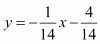(which is of the form y =mx +c)

∴Slope of the given line = -1/14

If the normal is parallel to the line, then we must have the slope of the normal being equal to the slope of the line.

When x = 2, y = 8 + 4 + 6 = 18.

When x = −2, y = − 8 − 4 + 6 = −6.

Therefore, there are two normals to the given curve with slope -1/14 and passing through the points (2, 18) and (−2, −6).

Thus, the equation of the normal through (2, 18) is given by,

And, the equation of the normal through (−2, −6) is given by,

Hence, the equations of the normals to the given curve (which are parallel to the given line) are x + 14y + 86 = 0.

Question22. Find the equations of the tangent and normal to the parabola y2 = 4ax at the point (at2, 2at).

Solution :
The equation of the given parabola is y2 = 4ax.

On differentiating y2 = 4ax with respect to x, we have:

Question23. Prove that the curves x =y2 and xy = k cut at right angles if 8k2 = 1. [Hint: Two curves intersect at right angle if the tangents to the curves at the point of intersection are perpendicular to each other.]

Solution :
The equations of the given curves are given as x =y2 and xy = k

Putting x =y2 in xy =k, we get:

Question24. Find the equations of the tangent and normal to the hyperbolaat the point (x0 ,y0)

Solution :
Differentiatingwith respect to x, we have:

Therefore, the slope of the tangent at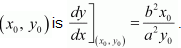Then, the equation of the tangent at (x0 ,y0is given by,

Question25. Find the equation of the tangent to the curve y = √3x – 2 which is parallel to the line 4x − 2y + 5 = 0.

Solution :
The equation of the given curve is y = √3x – 2

The slope of the tangent to the given curve at any point (x,y) is given by,

The equation of the given line is 4x − 2y + 5 = 0.

4x − 2y + 5 = 0⇒y = 2x + 5/2 (which is of the form y = mx + c)

∴Slope of the line = 2

Now, the tangent to the given curve is parallel to the line 4x − 2y − 5 = 0 if the slope of the tangent is equal to the slope of the line.

∴Equation of the tangent passing through the point(41/48, 3/4) is given by,

Hence, the equation of the required tangent is 48x – 24y = 23

Question26. The slope of the normal to the curve y = 2x2 + 3 sinx at x = 0 is

(A) 3

(B)1/3

(C) −3

(D) -1/3

Solution :
The equation of the given curve is y = 2x2 + 3 sinx

Slope of the tangent to the given curve at x = 0 is given by,

Hence, the slope of the normal to the given curve at x = 0 is

Question27. The liney =x + 1 is a tangent to the curve y2 = 4x at the point

(A) (1, 2)

(B) (2, 1)

(C) (1, −2)

(D) (−1, 2)

Solution :
The equation of the given curve is y2 = 4x

Differentiating with respect tox, we have:

Therefore, the slope of the tangent to the given curve at any point (x,y) is given by,

dy/dx = 2/y

The given line isy = x + 1 (which is of the form y =mx +c)

∴ Slope of the line = 1

The liney = x + 1 is a tangent to the given curve if the slope of the line is equal to the slope of the tangent. Also, the line must intersect the curve.

Thus, we must have:

Hence, the line y =x + 1 is a tangent to the given curve at the point (1, 2).

Exercise 6.4

Solve The Following Questions.

Question1. Using differentials, find the approximate value of each of the following up to 3 places of decimal:

(i) √25.3
(ii)√49.5
(iii)√0.6
(iv)(0.009)1/3
(v)(0.999)1/10
(vi) (15)1/4
(vii) (26)1/3
(viii) (255)1/4
(ix)
(82)1/4
(x)  (401)1/2
(xi) (0.0037)1/2
(xii) (26.57)1/3

(xiii) (81.5)1/4
(xiv) (3.968)3/2
(xv) (32.15)1/5
Solution :

(i) √25.3

(ii) √49.5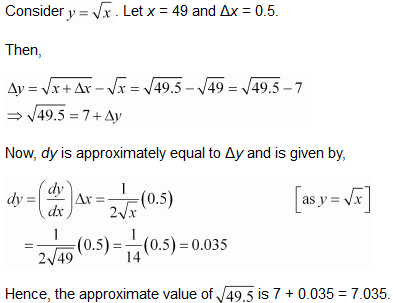(iii)  √0.6

(iv) (0.009)1/3

(v)(0.999)1/10

(vi) (15)1/4

(vii) (26)1/3

(viii) (255)1/4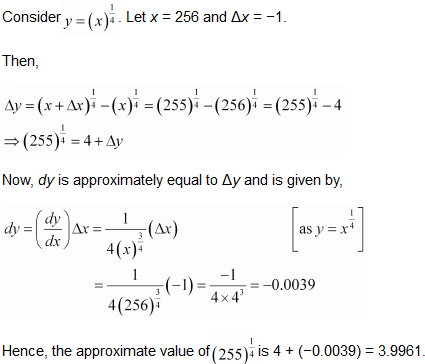(ix)(82)1/4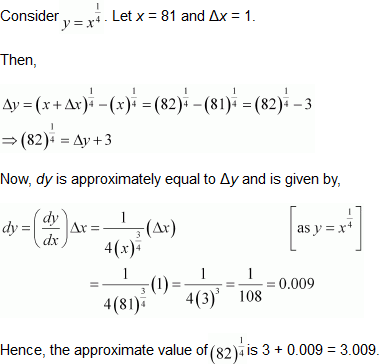(x) (401)1/2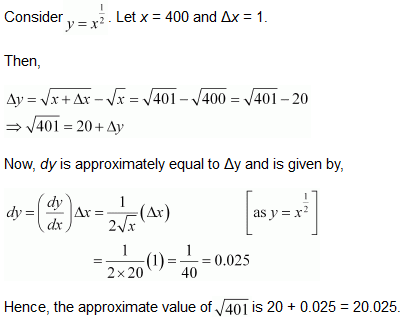(xi) (0.0037)1/2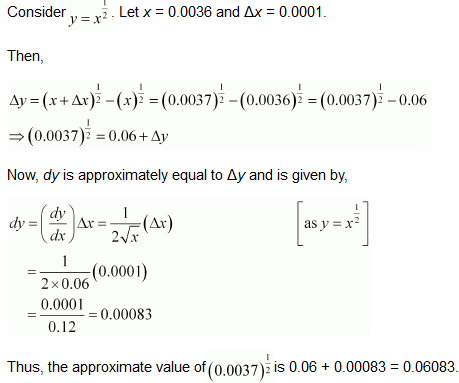(xii) (26.57)1/3

(xiii) (81.5)1/4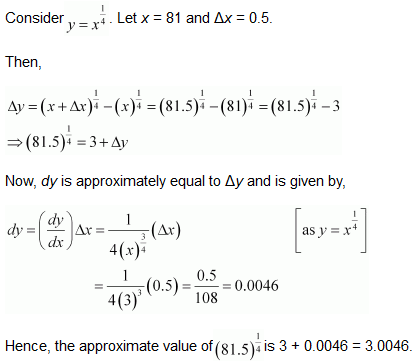(xiv)(3.968)3/2

(xv) (32.15)1/5

Question 2. Find the approximate value off (2.01), where f(x) = 4x2 + 5x + 2
Solution :

Hence, the approximate value of f (2.01) is 28.21.

Question 3. Find the approximate value of f (5.001), wheref (x) =x3 − 7x2 + 15.
Solution :

Hence, the approximate value of f (5.001) is −34.995.

Question 4. Find the approximate change in the volume of a cube of side x meters caused by increasing the side by 1%.
Solution :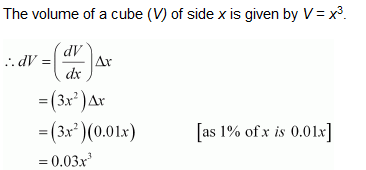Hence, the approximate change in the volume of the cube is 0.03x3 m3.

Question 5. Find the approximate change in the surface area of a cube of side x meters caused by decreasing the side by 1%.
Solution :

The surface area of a cube (S) of side x is given by S = 6x2.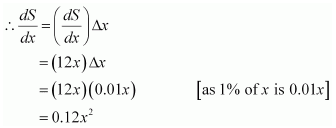Hence, the approximate change in the surface area of the cube is 0.12x2 m2.

Question 6. If the radius of a sphere is measured as 7 m with an error of 0.02 m, then find the approximate error in calculating its volume.
Solution :

Let r be the radius of the sphere and Δr be the error in measuring the radius.

Then,

r = 7 m and Δr = 0.02 m

Now, the volume V of the sphere is given by,

Hence, the approximate error in calculating the volume is 3.92 π m3.

Question 7. If the radius of a sphere is measured as 9 m with an error of 0.03 m, then find the approximate error in calculating its surface area.
Solution :

Let be the radius of the sphere and Δr be the error in measuring the radius.

Then,

r = 9 m and Δr = 0.03 m

Now, the surface area of the sphere (S) is given by,

S = 4πr2

Hence, the approximate error in calculating the surface area is 2.16π m2.

Question 8. Iff (x) = 3x+ 15x + 5, then the approximate value of f (3.02) is
A. 47.66
B. 57.66
C. 67.66
D.77.66
Solution :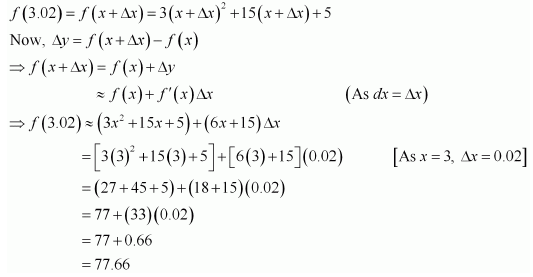Therefore, option (D) is correct.

Question 9. The approximate change in the volume of a cube of sidex metres caused by increasing the side by 3% is
A. 0.06x3 m3
B. 0.6x3 m3
C. 0.09x3 m3
D.0.9x3 m3
Solution :

The volume of a cube (V) of side x is given by V = x3.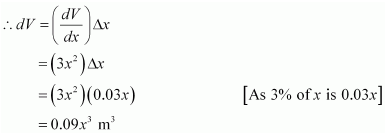Therefore, option (C) is correct.

### Solve The Following Questions.

Question1. Find the maximum and minimum values, if any, of the following functions given by

(i) f(x) = (2x − 1)2 + 3

(ii) f(x) = 9x2 + 12x + 2

(iii) f(x) = −(x − 1)2 + 10

(iv) g(x) =x3 + 1

Solution :
(i) The given function is f(x) = (2x − 1)2 + 3.

It can be observed that (2x− 1)2 ≥ 0 for every x∈R.

Therefore, f(x) = (2x − 1)2 + 3 ≥ 3 for every x∈R.

The minimum value of f is attained when 2x − 1 = 0.

2x − 1 = 0⇒ x = 1/2

∴Minimum value of f =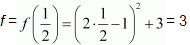= 3

Hence, functionf does not have a maximum value.

(ii) The given function is f(x) = 9x2 + 12x + 2 = (3x + 2)2 − 2.

It can be observed that (3x + 2)2 ≥ 0 for every x∈R.

Therefore, f(x) = (3x + 2)2 − 2 ≥ −2 for every x∈R.

The minimum value of f is attained when 3x + 2 = 0.

3x + 2 = 0⇒ x = -2/3

∴Minimum value off =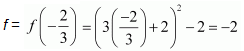Hence, functionf does not have a maximum value.

(iii) The given function is f(x) = − (x − 1)2 + 10.

It can be observed that (x − 1)2 ≥ 0 for every x∈R.

Therefore,f(x) = − (x − 1)2 + 10 ≤ 10 for every x∈R.

The maximum value off is attained when (x − 1) = 0.

(x − 1) = 0⇒x = 1

∴Maximum value off = f(1) = − (1 − 1)2 + 10 = 10

Hence, functionf does not have a minimum value.

(iv) The given function isg(x) =x3 + 1.

Hence, functiong neither has a maximum value nor a minimum value.

Question2. Find the maximum and minimum values, if any, of the following functions given by

(i) f(x) = |x + 2| − 1

(ii) g(x) = − |x + 1| + 3

(iii) h(x) = sin(2x) + 5

(iv) f(x) = |sin 4x + 3|

(v) h(x) =x+ 1,x ∈ (−1, 1)

Solution :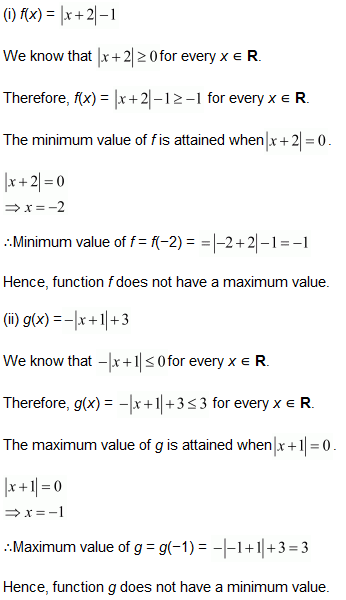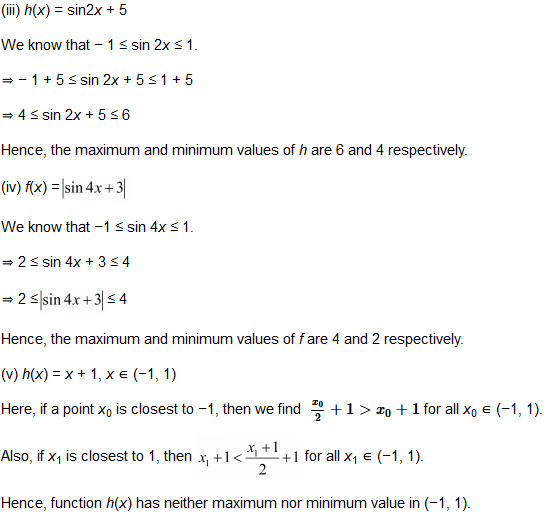.

Question3. Find the local maxima and local minima, if any, of the following functions. Find also the local maximum and the local minimum values, as the case may be:

(i).f(x) =x2

(ii).g(x) =x3 − 3x

(iii) h(x) = sinx + cosx, 0 < x < π/2

(iv) f(x) = sinx − cosx, 0 <x < 2π

(v) f(x) =x3 − 6x2+ 9x + 15

(vi)

(vii)

(viii)

Solution :

Question4. Prove that the following functions do not have maxima or minima:

(i) f(x) =ex

(ii) g(x) = logx

(iii) h(x) =x3 +x+ x + 1

Solution :
We have,

f(x) = ex

∴ f'(x) = ex

Now, if f'(x) = 0. But, the exponential function can never assume 0 for any value ofx.

Therefore, there does not existc∈R such that f'(c) = 0

Hence, functionf does not have maxima or minima.

We have,

g(x) = logx

∴ g'(x) = 1/x

Since log xnis defined for a positive number x, g'(x) > 0 for any x

Therefore, there does not existc∈R such that g'(c) = 0

Hence, functiong does not have maxima or minima.

We have,

h(x) =x3 +x2 +x + 1

h'(x) =3x2 +2x + 1

Now,

h(x) = 0 ⇒ 3x2 + 2x + 1 = 0⇒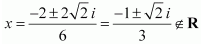Therefore, there does not exist c∈R such that  h'(c) = 0

Hence, functionh does not have maxima or minima.

Question5. Find the absolute maximum value and the absolute minimum value of the following functions in the given intervals:

(i)

(ii)

(iii)

(iv)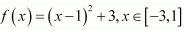Solution :
(i) The given function isf(x) = x3.

f'(x) = 3x2

Now, f'(x) = 0 ⇒  x =  0

Then, we evaluate the value off at critical point x = 0 and at end points of the interval [−2, 2].

f(0) = 0

f(−2) = (−2)3 = −8

f(2) = (2)3 = 8

Hence, we can conclude that the absolute maximum value off on [−2, 2] is 8 occurring atx = 2. Also, the absolute minimum value off on [−2, 2] is −8 occurring at x = −2.

(ii) The given function is f(x) = sinx + cosx.

Then, we evaluate the value off at critical point π/4 and at the end points of the interval [0, π].

Hence, we can conclude that the absolute maximum value off on [0, π] is√2  occurring atx = π4 and the absolute minimum value off on [0, π] is −1 occurring atx = π.

Then, we evaluate the value off at critical pointx = 4 and at the end points of the interval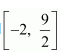.

Hence, we can conclude that the absolute maximum value off onis 8 occurring atx = 4 and the absolute minimum value off onis −10 occurring atx = −2.

Hence, we can conclude that the absolute maximum value off on [−3, 1] is 19 occurring atx = −3 and the minimum value off on [−3, 1] is 3 occurring atx = 1.

Question6. Find the maximum profit that a company can make, if the profit function is given by

p(x) = 41 − 72x − 18x2

Solution :
The profit function is given as p (x) = 41 − 72x− 18x2.

∴ p'(x)=−72−36x

⇒ x=−7236 =−2

Also, p”(−2)=−36 <0 By second derivative test, x=−2

is the point of local maxima of p.

∴ Maximum profit=p(−2)

=41−72(−2)−18(−2)2

=41+144−72=113

Hence, the maximum profit that the company can make is 113 units. The solution given in the book has some error. The solution is created according to the question given in the book.

Question7. Find both the maximum value and the minimum value of  3x− 8x3 + 12x2 − 48x + 25 on the interval [0, 3]

Solution :
Let f(x) = 3x− 8x+ 12x2 − 48x + 25.

Now,f'(x) = 0 gives x = 2 or x2+ 2 = 0 for which there are no real roots.

Therefore, we consider only x = 2∈[0, 3].

Now, we evaluate the value off at critical pointx = 2 and at the end points of the interval [0, 3].

Hence, we can conclude that the absolute maximum value offon [0, 3] is 25 occurring atx= 0 and the absolute minimum value off at [0, 3] is − 39 occurring atx = 2.

Question8. At what points in the interval [0, 2π], does the function sin 2x attain its maximum value?

Solution :
Letf(x) = sin 2x.

Question9. What is the maximum value of the function sinx + cosx?

Solution :
Let f(x) = sinx + cosx.

Now,f”(x) will be negative when (sinx + cosx) is positive i.e., when sin xand cosxare both positive. Also, we know that sinx and cosx both are positive in the first quadrant. Then,f”(x) will be negative when x ∊ (0, π/2).

Thus, we consider x = π/4

∴By second derivative test,f will be the maximum at x = π/4 and the maximum value of f is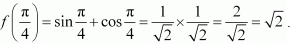.

Question10. Find the maximum value of 2x3 − 24x + 107 in the interval [1, 3]. Find the maximum value of the same function in [−3, −1].

Solution :
Let f(x) = 2x3 − 24x + 107.

We first consider the interval [1, 3].

Then, we evaluate the value off at the critical pointx = 2∈ [1, 3] and at the end points of the interval [1, 3].

f(2) = 2(8) − 24(2) + 107 = 16 − 48 + 107 = 75

f(1) = 2(1) − 24(1) + 107 = 2 − 24 + 107 = 85

f(3) = 2(27) − 24(3) + 107 = 54 − 72 + 107 = 89

Hence, the absolute maximum value off(x) in the interval [1, 3] is 89 occurring atx = 3.

Next, we consider the interval [−3, −1].

Evaluate the value off at the critical pointx = −2∈ [−3, −1] and at the end points of the interval [1, 3].

f(−3) = 2 (−27) − 24(−3) + 107 = −54 + 72 + 107 = 125

f(−1) = 2(−1) − 24 (−1) + 107 = −2 + 24 + 107 = 129

f(−2) = 2(−8) − 24 (−2) + 107 = −16 + 48 + 107 = 139

Hence, the absolute maximum value off(x) in the interval [−3, −1] is 139 occurring atx = −2.

Question11. It is given that atx = 1, the function x4− 62x2 +ax + 9 attains its maximum value, on the interval [0, 2]. Find the value of a.

Solution :
Let f(x) =x4 − 62x2 +ax + 9.

Hence, the value ofa is 120.

Question12. Find the maximum and minimum values ofx + sin 2x on [0, 2π].

Solution :
Let f(x) =x + sin 2x.

Hence, we can conclude that the absolute maximum value off(x) in the interval [0, 2π] is 2π occurring atx = 2π and the absolute minimum value off(x) in the interval [0, 2π] is 0 occurring atx = 0.

Question13. Find two numbers whose sum is 24 and whose product is as large as possible.

Solution :
Let one number be x. Then, the other number is (24 −x).

Let P(x) denote the product of the two numbers. Thus, we have:

∴By second derivative test,x = 12 is the point of local maxima of P. Hence, the product of the numbers is the maximum when the numbers are 12 and 24 − 12 = 12.

Question14. Find two positive numbers x andy such that x +y = 60 and xy3 is maximum.

Solution :
The two numbers arex andy such that x +y = 60.

⇒y = 60 −x

Let f(x) =xy3.

∴By second derivative test,x= 15 is a point of local maxima off. Thus, functionxy3 is maximum whenx = 15 andy = 60 − 15 = 45.

Hence, the required numbers are 15 and 45.

Question15. Find two positive numbersxandysuch that their sum is 35 and the product x2y5 is a maximum

Solution :
Let one number bex. Then, the other number is y = (35 −x).

Let P(x) =x2y5. Then, we have:

∴ By second derivative test, P(x) will be the maximum when x = 10 and y = 35 − 10 = 25.

Hence, the required numbers are 10 and 25.

Question16. Find two positive numbers whose sum is 16 and the sum of whose cubes is minimum.

Solution :
Let one number be x. Then, the other number is (16 −x).

Let the sum of the cubes of these numbers be denoted by S(x). Then,

∴ By second derivative test, x = 8 is the point of local minima of S.

Hence, the sum of the cubes of the numbers is the minimum when the numbers are 8 and 16 − 8 = 8.

Question17. A square piece of tin of side 18 cm is to made into a box without top, by cutting a square from each corner and folding up the flaps to form the box. What should be the side of the square to be cut off so that the volume of the box is the maximum possible?

Solution :
Let the side of the square to be cut off be x cm. Then, the length and the breadth of the box will be (18 − 2x) cm each and the height of the box is x cm.

Therefore, the volume V(x) of the box is given by,

∴ By second derivative test,x = 3 is the point of maxima of V.

Hence, if we remove a square of side 3 cm from each corner of the square tin and make a box from the remaining sheet, then the volume of the box obtained is the largest possible.

Question18. A rectangular sheet of tin 45 cm by 24 cm is to be made into a box without top, by cutting off square from each corner and folding up the flaps. What should be the side of the square to be cut off so that the volume of the box is the maximum possible?

Solution :
Let the side of the square to be cut off be x cm. Then, the height of the box isx, the length is 45 − 2x, and the breadth is 24 − 2x.

Therefore, the volumeV(x) of the box is given by,

∴By second derivative test,x = 5 is the point of maxima.

Hence, the side of the square to be cut off to make the volume of the box maximum possible is 5 cm.

Question19. Show that of all the rectangles inscribed in a given fixed circle, the square has the maximum area.

Solution :
Let a rectangle of lengthl and breadthb be inscribed in the given circle of radiusa.

Then, the diagonal passes through the centre and is of length 2a cm.

∴By the second derivative test, when l = √2a , then the area of the rectangle is the maximum.

Since l = b = √2a, the rectangle is a square.

Hence, it has been proved that of all the rectangles inscribed in the given fixed circle, the square has the maximum area.

Question20. Show that the right circular cylinder of given surface and maximum volume is such that is heights is equal to the diameter of the base.

Solution :
Letr andh be the radius and height of the cylinder respectively.

Then, the surface area (S) of the cylinder is given by,

Hence, the volume is the maximum when the height is twice the radius i.e., when the height is equal to the diameter.

Question21. Of all the closed cylindrical cans (right circular), of a given volume of 100 cubic centimetres, find the dimensions of the can which has the minimum surface area?

Solution :
Letr andh be the radius and height of the cylinder respectively.

Then, volume (V) of the cylinder is given by,

Question22. A wire of length 28 m is to be cut into two pieces. One of the pieces is to be made into a square and the other into a circle. What should be the length of the two pieces so that the combined area of the square and the circle is minimum?

Solution :
Let a piece of lengthl be cut from the given wire to make a square.

Then, the other piece of wire to be made into a circle is of length (28 −l) m.

Now, side of square = l/4

Letr be the radius of the circle. Then, 2πr = 28 – ⇒ r = 1/2π(28  – l)

The combined areas of the square and the circle (A) is given by,

Question23. Prove that the volume of the largest cone that can be inscribed in a sphere of radius R is 8/27 of the volume of the sphere.

Solution :
Letr andh be the radius and height of the cone respectively inscribed in a sphere of radius R.

Question24. Show that the right circular cone of least curved surface and given volume has an altitude equal to √2 time the radius of the base.

Solution :
Let randh be the radius and the height (altitude) of the cone respectively.

Then, the volume (V) of the cone is given as: V =13πr2h ⇒ h =3Vπr2

The surface area (S)of the cone is given by,

S = πrl (wherel is the slant height)

Hence, for a given volume, the right circular cone of the least curved surface has an altitude equal to√2 times the radius of the base.

Question25. Show that the semi-vertical angle of the cone of the maximum volume and of given slant height is tan-1 √2

Solution :
Letθ be the semi-vertical angle of the cone.

It is clear that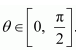Let r,h, and l be the radius, height, and the slant height of the cone respectively.

The slant height of the cone is given as constant.

Now,r =l sinθ and h =l cosθ

The volume (V) of the cone is given by,

V=1/3πr2h =1/3π (l2sin2θ)(lcosθ)

=1/3πl3 sinθ cosθ

⇒dV/dθ =l3π/3 [sin2θ (−sinθ) + cosθ(2sinθ cosθ)]

=l3π / 3[−sin3θ+2sinθcos2θ]

⇒d2V / dθ2 =l3π / 3 [−3sin2θ cosθ + 2cos3θ− 4sin2θ cosθ ]

=l3π/3 [2cos3θ − 7sin2θ cosθ]

∴By second derivative test, the volume (V) is the maximum when θ = tan-1 √2

Hence, for a given slant height, the semi-vertical angle of the cone of the maximum volume is tan-1 √2.

Question26. Show that semi-vertical angle of right circular cone of given surface area and maximum volume is sin-1 (1/3)

Solution :
Let r be the radius, l be the slant height and h be the height of the cone of given surface area,S.

Also, let α be the semi-vertical angle of the cone.

Question27. The point on the curve x2 = 2y which is nearest to the point (0, 5) is

(A) (2√2 , 4)

(B) (2√2 , 0)

(C) (0, 0)

(D) (2, 2)

Solution :
Equation of the curve is  x2 = 2y

For each value of x, the position of the point will be(x, x2/2)

Let P (x, x2/2) be any point on the curve (i), then according to question,

Distance between given point (0, 5) and

Therefore, option (A) is correct.

Question28. For all real values ofx, the minimum value ofis

(A) 0

(B) 1

(C) 3

(D) 1/3

Solution :
Let f =

∴By second derivative test,f is the minimum atx= 1 and the minimum value is given by f(1) = 1-1+1/1+1+1 = 1/3

Question29. The maximum value ofis

(A)(1/3)1/3

(B)1/2

(C) 1

(D) 0

Solution :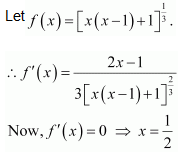Then, we evaluate the value off at critical point x = 1/2 and at the end points of the interval [0, 1] {i.e., atx = 0 andx = 1}.

Hence, we can conclude that the maximum value off in the interval [0, 1] is 1.

### Solve The Following Questions.

Question1. Using differentials, find the approximate value of each of the following:

(a) (17/81)1/4

(b) (33)1/5

Solution :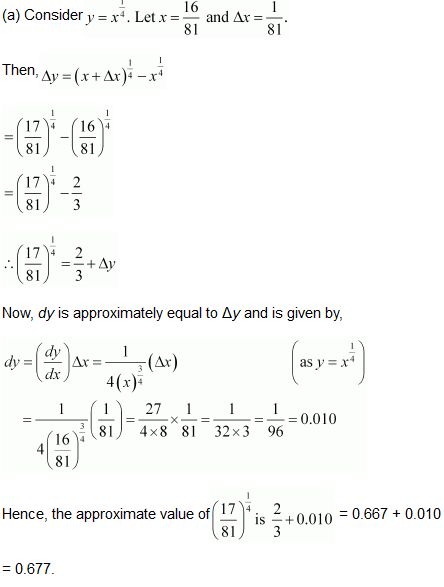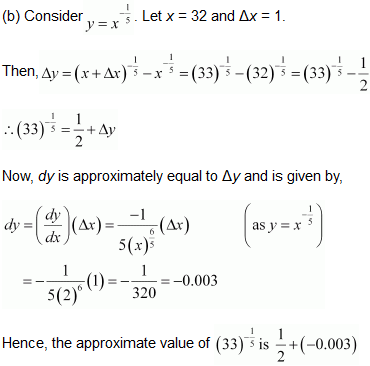=  0.497

Question2. Show that the function given by f(x) = logx / x  has maximum value at x = e

Solution :

Question3. The two equal sides of an isosceles triangle with fixed base b  are decreasing at the rate of 3 cm per second. How fast is the area decreasing when the two equal sides are equal to the base?

Solution :

Let ΔABC be isosceles where BC is the base of fixed length b.

Let the length of the two equal sides of ΔABC be a.

Therefore, the area is decreasing at the rate of √3b cm2/s.

Question4. Find the equation of the normal to the curve y2 = 4x at the point (1, 2).

Solution :
Equation of the curve is y2 = 4x……….(i)

5. Show that the normal at any point θ  to the curve x = a cosθ + a θ sin θ, y = a sin θ – aθ cos θ is at a constant distance from the origin.

Solution :
We have x = a cos θ + a θ sin θ.

Hence, the perpendicular distance of the normal from the origin is constant.

Question6. Find the intervals in which the function f given by   is (i) increasing (ii) decreasing.

Solution :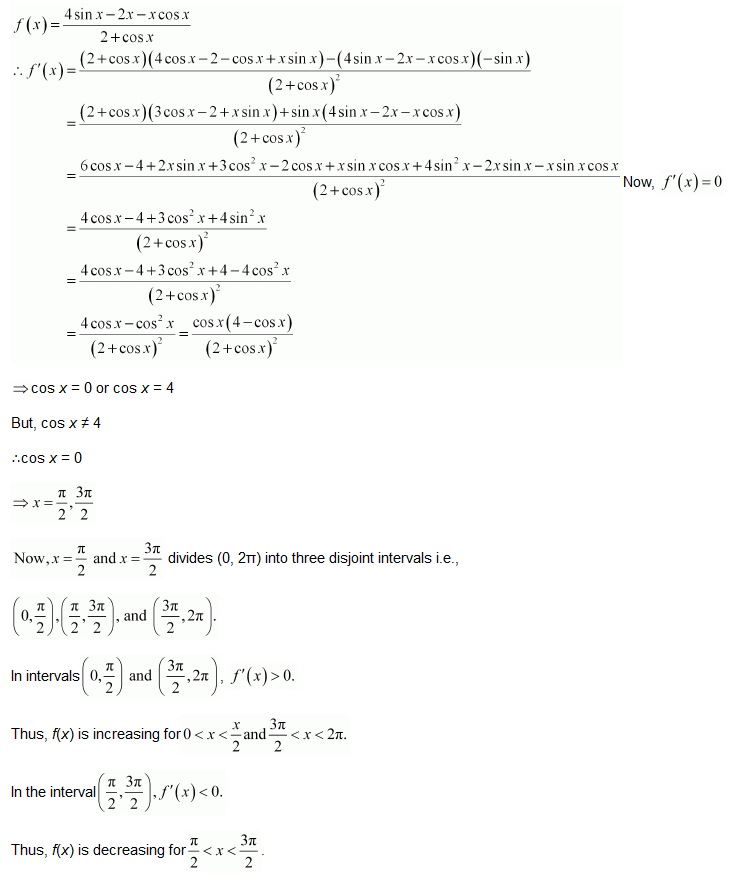Question7. Find the intervals in which the function f given by   is (i) increasing (ii) decreasing.

Solution :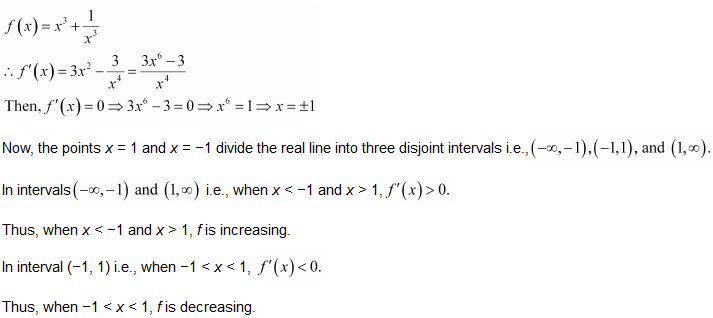Question8. Find the maximum area of an isosceles triangle inscribed in the ellipse   with its vertex at one end of the major axis.

Solution :
Equation of the ellipse is

Let the major axis be along the x −axis.

Let ABC be the triangle inscribed in the ellipse where vertex C is at (a, 0).

Since the ellipse is symmetrical with respect to the x−axis and y −axis, we can assume the coordinates of A to be (−x1y1) and the coordinates of B to be (−x1, −y1).

9. A tank with rectangular base and rectangular sides, open at the top is to be constructed so that its depth is 2 m and volume is 8 m3. If building of tank costs ` 70 per sq. meter for the base and ` 45 per square meter for sides. What is the cost of least expensive tank?

Solution :
Given: Depth of tank = 2 m

Let lb, and h represent the length, breadth, and height of the tank respectively.

Then, we have height (h) = 2 m

Volume of the tank = 8m3

Volume of the tank = l × b × h

∴ 8 = × b × 2

lb = 4, b = 4/l

Now, area of the base = lb = 4

Area of the 4 walls (A) = 2h (l + b)

Thus, by second derivative test, the area is the minimum when l = 2.

We have b = h = 2.

∴Cost of building the base = Rs 70 × (lb) = Rs 70 (4) = Rs 280

Cost of building the walls = Rs 2h (l + b) × 45 = Rs 90 (2) (2 + 2)

= Rs 8 (90) = Rs 720

Required total cost = Rs (280 + 720) = Rs 1000

Hence, the total cost of the tank will be Rs 1000.

Question10. The sum of the perimeter of a circle and square is k  where k  is some constant. Prove that the sum of their areas is least when the side of square is double the radius of the circle.

Solution :
Let r  be the radius of the circle and a  be the side of square.

Therefore, sum of areas is minimum when side of the square is double the radius of the circle.

Question11. A window is in the form of a rectangle surmounted by a semicircular opening. The total perimeter of the window is 10 m. Find the dimensions of the window to admit maximum light through the whole opening.

Solution :

Let and y be the length and breadth of the rectangular window.

Radius of the semicircular opening = x/2

It is given that the perimeter of the window is 10 m.

∴Area of the window (A) is given by,

Thus, when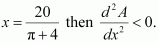Therefore, by second derivative test, the area is the maximum when length x = 20/π + 4m.

Hence, the required dimensions of the window to admit maximum light is given bylength = 20/π+4m and breadth = 10/π+4m.

Question12. A point on the hypotenuse of a triangle is at distances a and b  from the sides of the triangle. Show that the maximum length of the hypotenuse is

Solution :

Let ΔABC be right-angled at B. Let AB = x and BC = y.

Let P be a point on the hypotenuse of the triangle such that P is at a distance of and b from the sides AB and BC respectively.

Let ∠C = θ.

We have,

AC = √x2 + y2

Now,

PC = cosec θ

And, AP = a sec θ

∴AC = AP + PC

⇒ AC = b cosec θ + a sec θ … (1)

Therefore, by second derivative test, the length of the hypotenuse is the maximum when tan θ(b/a) 1/3

Now, when tan θ(b/a) 1/3, we have:

Hence, the maximum length of the hypotenuses is

Question13. Find the points at which the function f  given by f(x) = (x – 2)4 (x + 1) 3has:

(i) local maxima

(ii) local minima

(iii) point of inflexion.

Solution :
Given: f(x) = (x – 2)4 (x + 1) 3

Now, for values of close to 2/7 and to the left of  2/7, f'(x) > 0. Also, for values of x close to 2/7  and to the right of 2/7, f'(x) < 0

Thus, x = 2/7 is the point of local maxima.

Now, for values of x close to 2 and to the left of 2, f'(x) < 0 Also, for values of x close to 2 and to the right of 2, f'(x) > 0

Thus, x = 2 is the point of local minima.

Now, as the value of x varies through −1,f'(x) does not changes its sign.

Thus, x = −1 is the point of inflexion.

Question14. Find the absolute maximum and minimum values of the function f given by f(x) = cos2 x + sin x , x ∊ [0,π ]

Solution :
Given:f(x) = cos2 x + sin x  ……….(i)

Now, evaluating the value of at critical points  x = π/2 and x = π/6 and at the end points of the interval [0,π ] (i.e., at x = 0 and x = π), we have:

Hence, the absolute maximum value of f is 5/4 occurring at x = π/6  and the absolute minimum value of f is 1 occurring at x = 0, π/2, and π.

Question15. Show that the altitude of the right circular cone of maximum volume that can be inscribed in a sphere of radius r is 4r/3

Solution :
Let r  be the radius of base of cone and h  be the height of the cone inscribed in a sphere of radius

The volume (V) of the cone is given by,

V = 1/3πR2h

Now, from the right triangle BCD, we have:

BC = √r2 – R2

h = r+√r2 – R2

Hence, it can be seen that the altitude of the right circular cone of maximum volume that can be inscribed in a sphere of radius r is 4r/3.

Question16. Let f be a function defined on [ab] such that f ‘(x) > 0, for all x ∈ (ab). Then prove that f is an increasing function on (ab).

Solution :

Let x1, x2∈(a,b) such that x1<x2.

Consider the sub-interval [x1, x2]. Since f(x) is differentiable on (a, b) and [x1, x2]⊂(a,b).

Therefore, f(x) is continous on [x1, x2] and differentiable on (x1, x2).

By the Lagrange’s mean value theorm, there exists c∈(x1, x2) such that

f'(c)=f(x2)-f(x1)x2-x1          …(1)

Since f'(x) ) > 0 for all x∈(a,b), so in particular, f'(c) > 0.

f'(c)>0⇒f(x2)-f(x1)x2-x1>0          [Using (1)]

⇒f(x2)-f(x1)>0          [∵ x2-x1>0 when x1<x2]

⇒f(x2)>f(x1)⇒f(x1)<f(x2)

Since x1, x2 are arbitrary points in (a,b).

Therefore, x1<x2⇒f(x1)<f(x2) for all x1,x2∈(a, b)

Hence,f(x) is increasing on (a,b).

Question17. Show that the height of the cylinder of maximum volume that can be inscribed in a sphere of radius R is 2R/√3 Also find the maximum volume.

Solution :

A sphere of fixed radius (R) is given.

Let r and h be the radius and the height of the cylinder respectively.

From the given figure, we have h = 2√R2 – r2

The volume (V) of the cylinder is given by,

Now, it can be observed that at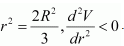∴The volume is the maximum when r2 = 2R2/3

When r2 = 2R2/3, the height of the cylinder is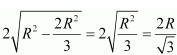Hence, the volume of the cylinder is the maximum when the height of the cylinder is 2R/√3

Question18. Show that the height of the cylinder of greatest volume which can be inscribed in a right circular cone of height h and having semi-vertical angle α  is one-third that of the cone and the greatest volume of the cylinder is 4/27πhtan2α.

Solution :

The given right circular cone of fixed height (h) and semi-vertical angle (α) can be drawn as:

Here, a cylinder of radius R and height H is inscribed in the cone.

Then, ∠GAO = α, OG = r, OA = h, OE = R, and CE = H.

We have,

h tan α

Now, since ΔAOG is similar to ΔCEG, we have:

Now, the volume (V) of the cylinder is given by,

∴By second derivative test, the volume of the cylinder is the greatest when

Thus, the height of the cylinder is one-third the height of the cone when the volume of the cylinder is the greatest.

Now, the maximum volume of the cylinder can be obtained as:

Choose the correct answer in the Exercises 19 to 24:

Question19. A cylindrical tank of radius 10 m is being filled with wheat at the rate of 314 cubic meter per hour. Then the depth of wheat is increasing at the rate of:

(A) 1 m/h

(B) 0.1 m/h

(C) 1.1 m/h

(D) 0.5 m/h

Solution :

Let r be the radius of the cylinder.

Then, volume (V) of the cylinder is given by,

Differentiating with respect to time t, we have:

The tank is being filled with wheat at the rate of 314 cubic metres per hour.

Thus, we have:

Hence, the depth of wheat is increasing at the rate of 1 m/h.

Question20. The slope of the tangent to the curve x = t2  + 3t – 8, y = 2t2  – 2t – 5 at the point (2,– 1) is

(A) 22/7

(B) 6/7

(C) 7/6

(D) -6/7

Solution :
The given curve is x = t2  + 3t – 8 and y = 2t2  – 2t – 5

The given point is (2, −1).

At x = 2, we have:

The common value of t is 2.

Hence, the slope of the tangent to the given curve at point (2, −1) is

Therefore, option (B) is correct.

Question21. The line y = mx + 1 is a tangent to the curve y2 = 4x if the value of m is

(A) 1

(B) 2

(C) 3

(D) 1/2

Solution :

The equation of the tangent to the given curve is y = mx + 1.

Now, substituting y = mx + 1 in y2 = 4x, we get:

Since a tangent touches the curve at one point, the roots of equation (i) must be equal.

Therefore, we have:

Discriminant = 0

Hence, the required value of m is 1.

Therefore, option (A) is correct.

Question22. The normal at the point (1, 1) on the curve 2y + x2 = 3 is

(A) x + y = 0

(B) x − = 0

(C) x + y + 1 = 0

(D) − y = 1

Solution :

The equation of the given curve is 2y + x2 = 3.

Differentiating with respect to x, we have:

The slope of the normal to the given curve at point (1, 1) is

Hence, the equation of the normal to the given curve at (1, 1) is given as:

⇒ y – 1 = 1(x – 1)

⇒ y – 1 = x – 1

⇒ x – y = 0

Therefore, option (B) is correct.

Question23. The normal to the curve x2 = 4y passing (1, 2) is

(A) x + y = 3

(B) x − y = 3

(C) x + = 1

(D) x − = 1

Solution :

The equation of the given curve is x2 = 4y.

Differentiating with respect to x, we have:

The slope of the normal to the given curve at point (hk) is given by,

∴Equation of the normal at point (hk) is given as:

Now, it is given that the normal passes through the point (1, 2).

Therefore, we have:

Since (hk) lies on the curve x2 = 4y, we have h2 = 4k.

⇒ k =h2/4

From equation (i), we have:

Hence, the equation of the normal is given as:

Therefore, option (A) is correct.

Question24. The points on the curve 9y2 = x3  where the normal to the curve make equal intercepts with axes are:

Solution :

The equation of the given curve is 9y2 = x3.

Differentiating with respect to x, we have:

The slope of the normal to the given curve at point (x1,y1) is

∴ The equation of the normal to the curve at (x1,y1) is

It is given that the normal makes equal intercepts with the axes.

Therefore, We have:

Also, the point(x1,y1)lies on the curve, so we have

9y12 = x13    …………………(ii)

From (i) and (ii), we have:

Therefore, option (A) is correct.

Get 30% off your first purchase!

X
error: Content is protected !!
Scroll to Top